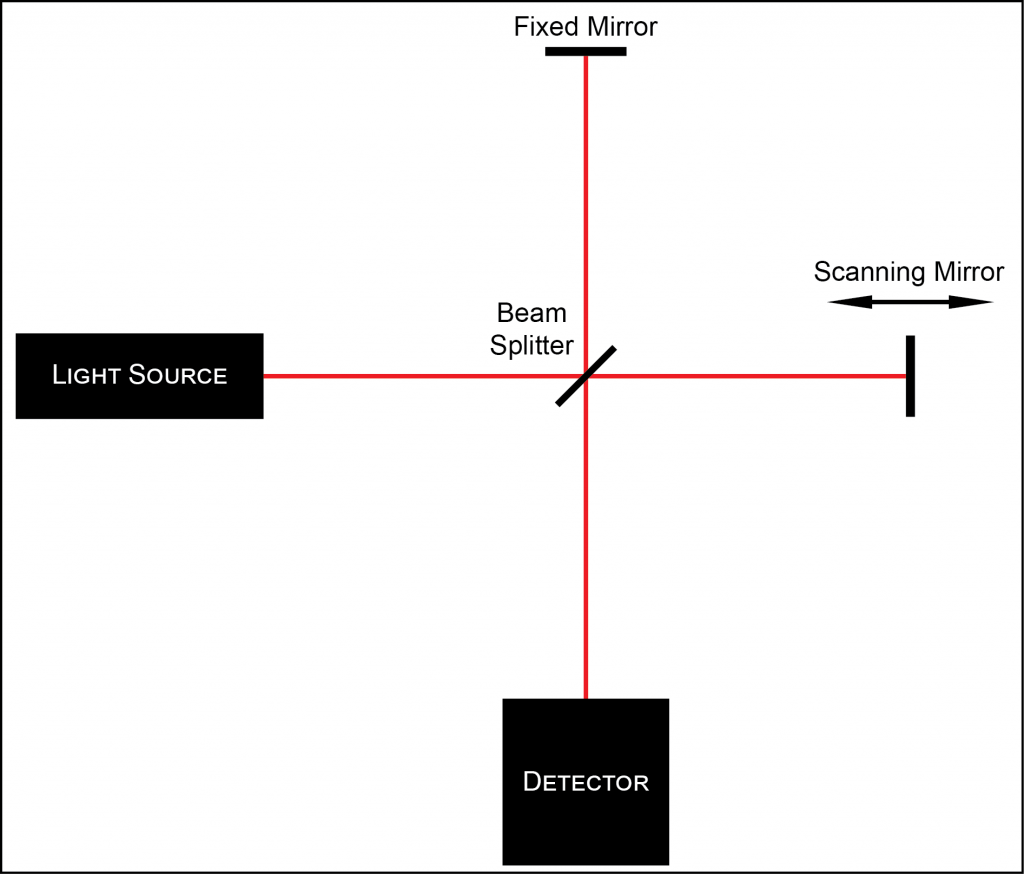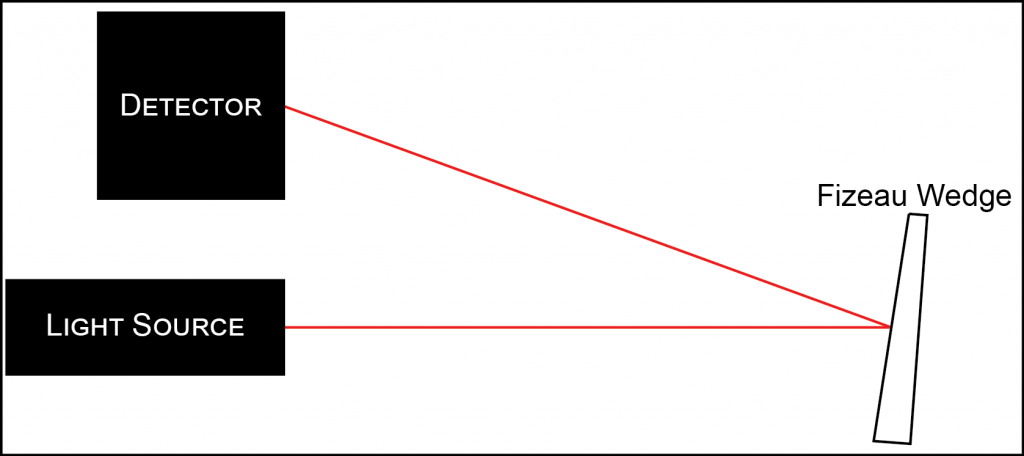## Question

What types of different wavelength meters are available and what are their accuracies?

Wavelength meters are devices that are able to accurately determine the wavelength of an input light source. They determine the input wavelength by sending the light through an interferometer, and analyzing the resulting interference pattern.

The two main types of interferometers that are used are the Michelson (scanning) and the Fizeau (static).

MICHELSON INTERFEROMETER
The scanning Michelson interferometer utilizes a beam splitter to decompose the incoming light into two paths. Each path then goes out to a mirror, which returns the light to the beam splitter. One mirror is static, while the other mirror is scanning (moving back and forth). The scanning mirror causes a difference in path lengths, which in turn causes an interference pattern once the light is recombined at the detector.Figure 1. Michelson Interferometer

This interference pattern has maxima and minima (bright and dark fringes), and the distance between the bright fringes is dependent on the wavelength.

 λ = 2d/N (1)

Where λ is the wavelength of the light being analyzed, d is the spacing between concurrent maxima, and N is the total number of maxima in the viewing window.

Currently, the highest accuracy available for Michelson models is 0.0001 nm (0.1 pm).

FIZEAU INTERFEROMETER
A basic, static Fizeau interferometer utilizes a wedge with a partially reflective front surface, and a fully reflective back surface. The front surface is offset by a small angle with respect to the back surface. Many wavelength meters available use multiple Fizeau interferometers to improve accuracy.Figure 2. Fizeau Interferometer

Again, the difference in path length (between the reflection from the front surface and the back surface) causes an interference pattern that is wavelength-dependent. By analyzing the resulting interference pattern, the wavelength of the incident light can be backed out. Some models do this by comparing to known spectra, while others model the signal mathematically to determine the wavelength.

Currently, the highest accuracy available for Fizeau models is 0.01 pm.

COMPARISON
There are differences in the two models aside from the type of interferometer utilized.

Michelson interferometers must have a reference laser for calibration built-in to the unit. Some models of Fizeau wavelength meters also offer this continuous calibration feature.

There are also differences in the types of measurements that can be taken with the different models. Pulsed lasers (up to 1 kHz rep rate) must be measured using a Fizeau, the Michelson can only support CW. CW lasers are able to be used with both configurations.

The wavelength range required is another differentiating factor. Michelson versions have a large range of wavelengths that can be used, from the UV (375 nm) up to the IR (12 µm). Fizeau versions generally work up to 1750 nm (1.75 µm).

As the wavelength changes, so does the accuracy of the measurement. Instrument-specific datasheets give the accuracy in different wavelength ranges, and vary from model to model.